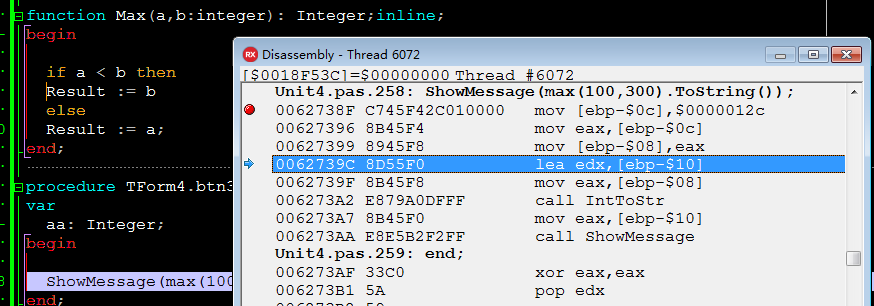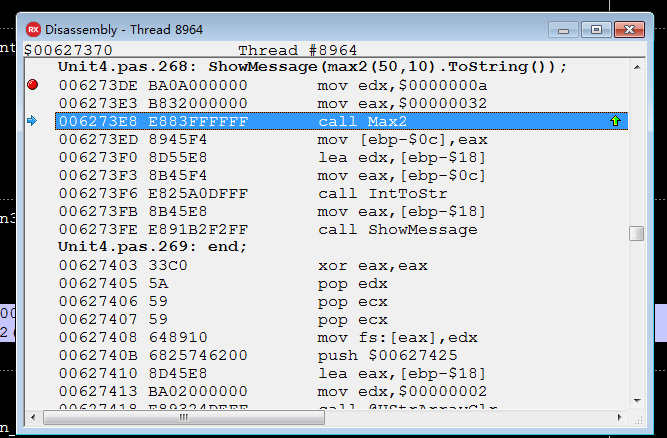# delphi 内联函数 inline

function Max(a,b:integer): Integer;inline;
begin

if a < b then
Result := b
else
Result := a;
end;

procedure TForm4.btn3Click(Sender: TObject);
var
aa: Integer;
begin

ShowMessage(max(100,300).ToString());
end;function Max2(a,b:integer): Integer;
begin

if a < b then
Result := b
else
Result := a;
end;